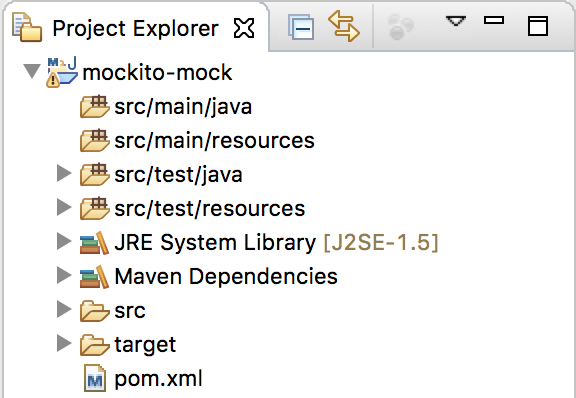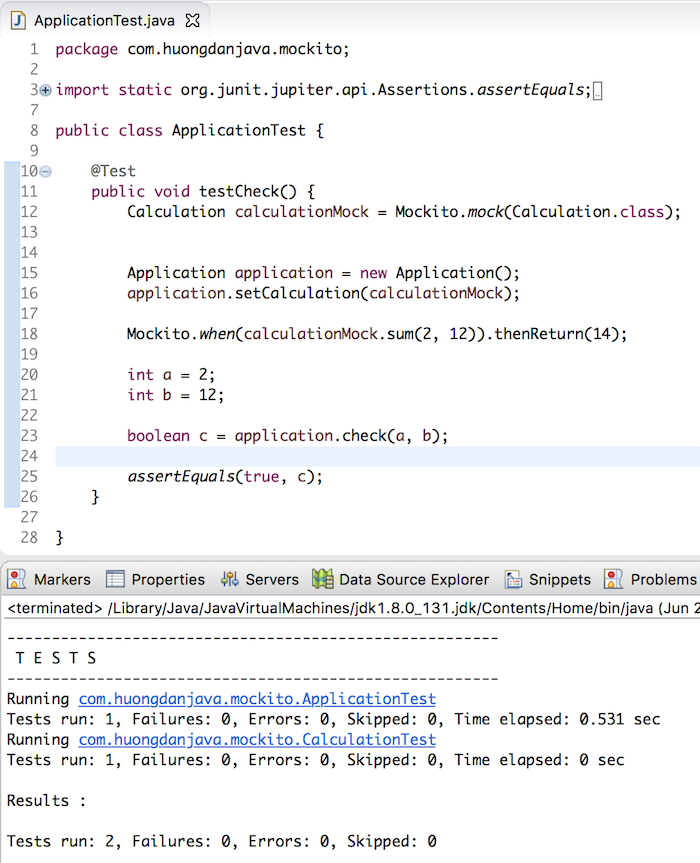# Overview about Mock in Unit Test

Unit Test is a tool that helps us to ensure that the code we write, reflects what we want accurately. In the process of working with the Unit Test, one of the problems we often encounter is that our code will call the methods of other classes, outside the class, the method we are writing. We can initialize those outer classes to call the method we want, but no one knows that the methods of those outer classes also call to other methods of other external classes. And the question is: whether it is important to pay attention to these external classes when we are testing the method of this class because those external classes also have their own Unit Tests.

The answer is no. The Unit Test is a test of small code, will have a Unit Test to ensure the logic for external dependencies, to ensure that the current code we have tested. In this case, the problem is that if the method we are calling to the method of the outer class is processed, how does the code call that method? How can the returns help us cover the logic in the Unit Test.

To solve this problem, in Unit Test, one concept is called Mock, which simulates the behavior of external classes to perform the behavior we expect. Then, the code that calls the external methods will return the value we expect. Because of controlling the code by ourself, the simulator still guarantees the quality of the code we are writing Unit Test. In this tutorial, I will show you all how to use Mock in the Unit Test with a popular Java Mocking library called Mockito.

First, I will create a new Maven project as an example:with Mockito and JUnit dependencies as follows:

With JUnit 5, to run Unit Test with Maven, we need to declare the maven-surefire-plugin plugin of Maven with the latest version as follows:

And compile source with target using Java 8 onwards:

Now that I’m going to create a small application that will allow users to pass in two numbers, we’ll compute those two numbers, which will return true if the result is greater than 10, otherwise false. The code will look like this:

We have a class to sum two numbers:

A main class of applications in which it has a method for checking the result of the computation and returns true, false based on the result:

The results are as follows:Now, we will write Unit Test for our classes.

I will create a class CalculationTest to test the class Calculation with the following content:

Unit Test for class Calculation, it is simple, right ? 😀

For the Unit Test of the Application class, the main() method is the method to run the application, so we do not need to test. Only check() method we need to test.

Normally, I can write Unit Test for this check() method as follows:

But if you look carefully, you will see the following code in the check() method of the Application class:

we tested in the above CalculationTest class, so we will not need to test again. The only thing we need to test in this check() method is just the return code.

Now, let’s apply Mock for Calculation class.

First, you need to use Mockito to create a Mock object for the Calculation class:

As you can see, here I have used the mock() static method of the Mockito class in the Mockito library to create a Mock object for the Calculation class. There are many overload mock() static methods in the Mockito class, depending on the needs you can use.

Once you have the Mock object of the Calculation class, you can set this mock object for use in the Application class as follows:

Now we will simulate the behavior of the Calculation object in the Application class using its Mock object.

Assuming now, I want to when the Application class calls the sum() method of two numbers 2 and 12 in the Calculation class, I will return 14 without regard to the logic of sum() method, then I will mock as follows:

You can return any value but make sure it’s the right expectation.

The entire testCheck() code will now look like this:

Result:If you now modify the mock code, the sum() method returns 8:

The results are as follows:

As you can see, using Mock in the Unit Test makes it possible to determine the result from external classes without regard to their handling. Just make sure the out code, right? 🙂